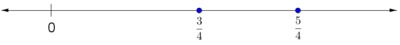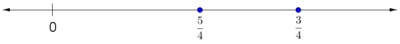# Comparing Fractions with the Same Denominator, Assessment Variation

Alignments to Content Standards: 3.NF.A.3.d

1. Choose each statement that is true.
1. $\frac34$ is greater than $\frac54$.
2. $\frac54$ is greater than $\frac34$.
3. $\frac34 \gt \frac54$.
4. $\frac34 \lt \frac54$.
5. $\frac54 \gt \frac34$.
6. $\frac54 \lt \frac34$.
7. None of these.

2. $\frac34$ and $\frac54$ are shown on the number line. Which is correct?

1.2.3. Neither of these.

## IM Commentary

This task is part of a joint project between Student Achievement Partners and Illustrative Mathematics to develop prototype machine-scorable assessment items that test a range of mathematical knowledge and skills described in the CCSSM and begin to signal the focus and coherence of the standards.

This task is part of a set of three assessment tasks for 3.NF.3d. This part of the standard is about comparing two fractions with the same numerator or the same denominator by reasoning about their size, and understanding that such comparisons are valid only when the fractions refer to the same whole.

• 3.NF Comparing Fractions with the Same Denominators asks students to compare fractions using words and symbols and then to correctly represent them on the number line. This and the next task are designed to distinguish an understanding of relative magnitude, proficiency with symbolic notation, and proficiency with the number line. For example, if a student correctly chooses the greater fraction and the correct placement on the number line but selects the wrong inequalities, we diagnose that student as having trouble with the symbolic representation but not with their understanding of the relative magnitude of the fractions.
• 3.NF Comparing Fractions with the Same Numerators is identical in structure to the first task.
• 3.NF Fraction Comparisons with Pictures asks students to select pictures that accurately represent the relative magnitude of two fractions. This task also requires that students recognize that in order to compare two fractions, they must refer to the same whole.

### Cognitive Complexity

##### Mathematical Content

Comparing fractions with common denominators should be as easy as comparing 5 oranges and 7 oranges: the greater numerator corresponds to the greater fraction because we are comparing different quantities of the same unit. Comparing fractions with a common numerator is more complex because the smaller denominator corresponds to the greater fraction. Thus, “3.NF Comparing Fractions with the Same Numerator” is more complex than “3.NF Comparing Fractions with the Same Denominator.”

"3.NF Fraction Comparisons with Pictures" requires students to compare two fractions with the same numerators and then select pictures from a set of choices that can be used to accurately represent the comparison. For the first two tasks in this set, students can choose any method or representation they like to compare the fractions. This task is more complex because students must evaluate pictures they did not draw themselves and must understand that the wholes (rather than the pieces) must be the same size.

##### Mathematical Practice

The third task partially assesses students' ability to construct viable arguments and critique the reasoning of others (MP 3). Students have to evaluate three different claims and choose the correct one; they also must identify pictures that could be used to support an argument about comparing two fractions.

##### Linguistic Demand

The linguistic demand for the first two tasks is low. The linguistic demand for the third task is medium for third grade.

##### Stimulus Material

The first two tasks require students to analyze both written and symbolic statements and select a number line. The third task requires students to analyze written statements, construct a symbolic statement, and select diagrams from several options. Thus, the stimulus material for all three tasks is complex.

##### Response Mode

The response mode for the first two tasks is multiple choice (select all) and so is not complex. The third task requires students to use a drop-down menu and to drag pictures on the screen, which gives this task a medium level of response mode complexity. Note that they can try different things without penalty and there is a reset option.

## Solution

a. $\frac54$ is greater than $\frac34$ is true.

$\frac34 \lt \frac54$ is true.

$\frac54 \gt \frac34$ is true.

b. The first number line is correct.

This is a 2-point item. There are four correct pieces in this item. Each selection of an incorrect statement negates the credit for one correct selection.

2 points: All four correct pieces are selected and no incorrect selections are made. 1 point: Two or three total correct selections are made

• The student selects the three correct statements, one incorrect statement, and the correct number line
• The student selects three correct statements and an incorrect number line
• The student only selects one correct statement and the correct number line.

0 points: Zero or one total correct selections are made. For example,

• The student selects six statements and the correct number line
• The student only selects two correct statements and an incorrect number line
• The student selects no statements and a correct number line.